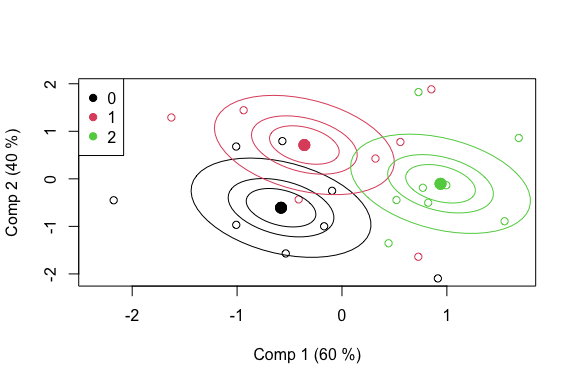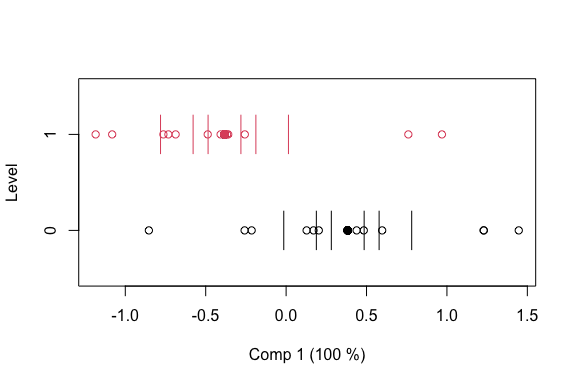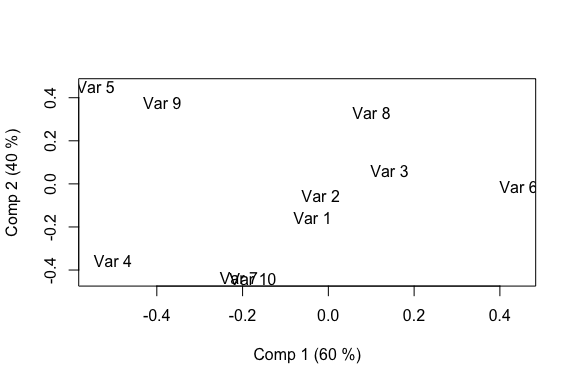# D. ASCA

library(multiblock)

# ANOVA Simultaneous Component Analysis – ASCA

The following example uses a simulated dataset for showcasing some of the possibilities of the ASCA method.

## Simulated data

Two categorical factors and a covariate are simulated together with a standard normal set of 10 responses.

set.seed(1)
dataset   <- data.frame(y = I(matrix(rnorm(24*10), ncol = 10)),
x = factor(c(rep(2,8), rep(1,8), rep(0,8))),
z = factor(rep(c(1,0), 12)), w = rnorm(24))
colnames(dataset$y) <- paste('Var', 1:10, sep = " ") rownames(dataset) <- paste('Obj', 1:24, sep = " ") str(dataset) #> 'data.frame': 24 obs. of 4 variables: #>$ y: 'AsIs' num [1:24, 1:10] -0.626 0.184 -0.836 1.595 0.33 ...
#>   ..- attr(*, "dimnames")=List of 2
#>   .. ..$: NULL #> .. ..$ : chr [1:10] "Var 1" "Var 2" "Var 3" "Var 4" ...
#>  $x: Factor w/ 3 levels "0","1","2": 3 3 3 3 3 3 3 3 2 2 ... #>$ z: Factor w/ 2 levels "0","1": 2 1 2 1 2 1 2 1 2 1 ...
#>  \$ w: num  0.707 1.034 0.223 -0.879 1.163 ...

## Formula interface

This ASCA implementation uses R’s formula interface for model specification. This means that the first argument is a formula with response on the left and design on the right, separated by a tilde operator, e.g. y ~ x + z or assessment ~ assessor + candy. The names in the formula refer to variables in a data.frame (or list). Separation with plus (+) adds main effects to the model, while separation by stars (*) adds main effects and interactions, e.g. y ~ x * z. Colons (:) can be used for explicit interactions, e.g. y ~ x + z + x:z. More complicated formulas exist, but only a simple subset is supported by asca.

## ASCA modelling

A basic ASCA model having two factors is fitted and printed as follows.

mod <- asca(y~x+z, data = dataset)
print(mod)
#> Anova Simultaneous Component Analysis fitted using 'lm' (Linear Model)
#> Call:
#> asca(formula = y ~ x + z, data = dataset)

## Scores

Scores for first factor are extracted and a scoreplot with confidence ellipsoids is produced.

sc <- scores(mod)
#>          Comp 1     Comp 2
#> Obj 1 0.9395791 -0.1039977
#> Obj 2 0.9395791 -0.1039977
#> Obj 3 0.9395791 -0.1039977
#> Obj 4 0.9395791 -0.1039977
#> Obj 5 0.9395791 -0.1039977
#> Obj 6 0.9395791 -0.1039977

scoreplot(mod, legendpos = "topleft", ellipsoids = "confidence")This is repeated for the second factor.

sc <- scores(mod, factor = "z")
#>           Comp 1
#> Obj 1 -0.3831621
#> Obj 2  0.3831621
#> Obj 3 -0.3831621
#> Obj 4  0.3831621
#> Obj 5 -0.3831621
#> Obj 6  0.3831621

scoreplot(mod, factor = "z", ellipsoids = "confidence")A basic loadingplot for the first factor is generated using graphics from the pls package.

lo <- loadings(mod)
loadingplot(mod, scatter = TRUE, labels = 'names')